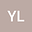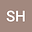Regularity Criterion for the Liquid Crystal Flow in $\mathbb{R}^3$}
••We obtain that a weak solution $(u,d)$ to the Liquid Crystal system is strong, if any two components of $u$ and $\nabla d$ satisfy Ladyzhenskaya-Prodi-Serrin’s condition, that is $$\|u^1\|_{L^{r,s}}+\|u^2\|_{L^{r,s}}+\|\nabla d\|_{L^{r,s}}\leq \infty, \qquad\frac{3}{r}+\frac{2}{s}\leq1.$$ We also prove that the velocity $u$ is bounded locally if any two comonents of $u$ and $\nabla d$ belong to $L^{6,\infty}$ and $u$ belongs to $L^{2+\delta}$ ($\delta>0$).